Request a Free Counselling Session from our Expert Mentor# NCERT Solution for Class 10th Maths Chapter 15 Probability

This chapter focuses on the fundamental concepts of probability, including its definition, types of events, and calculation of probability of events.
NCERT Solutions provided by Swastik Classes are designed to help students understand and solve the problems of this chapter in an easy and efficient manner. Our expert team has created the solutions in a step-by-step approach to enable students to understand the concepts thoroughly.
By using our solutions, students can improve their problem-solving skills and prepare well for their board exams. These solutions are prepared based on the latest CBSE guidelines and are strictly based on the NCERT textbook.
We hope our solutions will be beneficial to students in their academic journey and help them achieve their academic goals.

## Answers of Maths NCERT Solution for Class 10th Maths Chapter 15 Probability

Unit 15

Probability

Exercise 15.1

Question 1:

Complete the following statements:

1.    Probability of an event E + Probability of the event ‘not E’ =____.
2.    The probability of an event that cannot happen is ___. Such as event is called        _________.
3.    The probability of an event that is certain to happen is____. Such as event is

called____.

1.    The sum of the probabilities of all the elementary events of an experiment     is_______
2.      The probability of an event is greater than or equal to____ and less than or        equal to____.

1. 1
1. 0, impossible event
1. 1, sure event or certain event
1. 1
1. 0, 1

Question 2:

Which of the following experiments have equally likely outcomes? Explain.

1. A driver attempts to start a car. The car starts or does not start.
2. A player attempts to shoot a basketball. She/he shoots or misses the shot.
4. A baby is born. It is a boy or a girl.

1. It is not an equally likely event, as it depends on various factors such as whether the car will start or not. And factors for both the conditions are not the same.
1. It is not an equally likely event, as it depends on the player’s ability and there is no information given about that.
1. It is an equally likely event.
1. It is an equally likely event.

Question 3:

Why is tossing a coin considered to be a fair way of deciding which team should get the ball at the beginning of a football game?

When we toss a coin, the possible outcomes are only two, head or tail, which are equally likely outcomes. Therefore, the result of an individual toss is completely unpredictable.

Question 4:

Which of the following cannot be the probability of an event?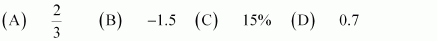Probability of an event (E) is always greater than or equal to 0. Also, it is always less than or equal to one. This implies that the probability of an event cannot be negative or greater than 1. Therefore, out of these alternatives, −1.5 cannot be a probability of an event.

Hence, (B)

Question 5:

If P(E) = 0.05, what is the probability of ‘not E’?

We know that,

Therefore, the probability of ‘not E’ is 0.95.

Question 6:

A bag contains lemon ﬂavoured candies only. Malini takes out one candy without looking into the bag. What is the probability that she takes out:

1. an orange ﬂavoured candy?
2. a lemon ﬂavoured candy?

1. The bag contains lemon ﬂavoured candies only. It does not contain any orange ﬂavoured candies. This implies that every time, she will take out only lemon ﬂavoured candies. Therefore, event that Malini will take out an orange ﬂavoured candy is an impossible event.

Hence, P (an orange ﬂavoured candy) = 0

1.   As the bag has lemon ﬂavoured candies, Malini will take out only lemon ﬂavoured candies. Therefore, event that Malini will take out a lemon ﬂavoured candy is a sure event.

P (a lemon ﬂavoured candy) = 1

*Question 7:

It is given that in a group of 3 students, the probability of 2 students not having the same birthday is 0.992. What is the probability that the 2 students have the same birthday?

Probability that two students are not having same birthday

Probability that two students are having same birthday

= 0.008

Question 8:

A bag contains 3 red balls and 5 black balls. A ball is drawn at random from the bag. What is the probability that the ball drawn is (i) red? (ii) not red?

1. Total number of balls in the bag = 8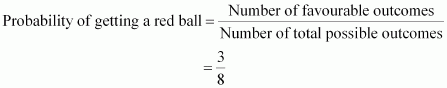1.   Probability of not getting red ball

= 1 − Probability of getting a red ball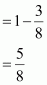Question 9:

A box contains 5 red marbles, 8 white marbles and 4 green marbles. One marble is taken out of the box at random. What is the probability that the marble taken out will be

(i) red? (ii) white? (iii) not green?

Total number of marbles = 5 + 8 + 4= 17

1. Number of red marbles = 5
1. Number of white marbles = 8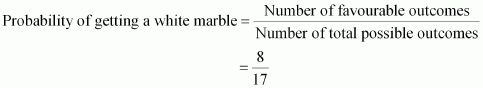1. Number of green marbles = 4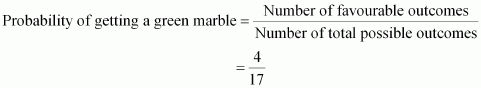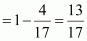Probability of not getting a green marble(Equation

Question 10:

A piggy bank contains hundred 50 p coins, ﬁfty Rs 1 coins, twenty Rs 2 coins and ten Rs 5 coins. If it is equally likely that one of the coins will fall out when the bank is turned upside down, what is the probability that the coin

1. Will be a 50 p coin?
2. Will not be a Rs.5 coin?

Total number of coins in a piggy bank = 100 + 50 + 20 + 10= 180

1. Number of 50 p coins = 100
1. Number of Rs 5 coins = 10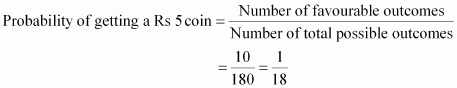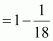Probability of not getting a Rs 5 coin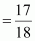*Question 11:

Gopi buys a ﬁsh from a shop for his aquarium. The shopkeeper takes out one ﬁsh at random from a tank containing 5 male ﬁsh and 8 female ﬁsh (see the given ﬁgure). What is the probability that the ﬁsh taken out is a male ﬁsh?Total number of ﬁshes in a tank

= Number of male ﬁshes + Number of female ﬁshes

= 5 + 8 = 13

Question 12:

A game of chance consists of spinning an arrow which comes to rest pointing at one of the numbers 1, 2, 3, 4, 5, 6, 7, 8 (see the given ﬁgure), and these are equally likely outcomes. What is the probability that it will point at

1. 8?
2. an odd number?
3. a number greater than 2?
4. a number less than 9?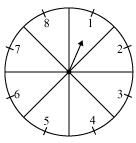Total number of possible outcomes = 8

(i)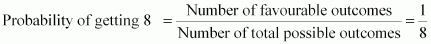1. Total number of odd numbers on spinner = 4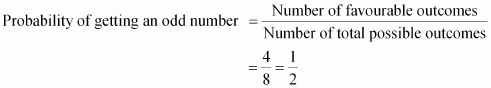1. The numbers greater than 2 are 3, 4, 5, 6, 7, and 8.

Therefore, total numbers greater than 2 = 6

1. The numbers less than 9 are 1, 2, 3, 4, 6, 7, and 8.

Therefore, total numbers less than 9 = 8

Probability of getting a number less than 9

*Question 13:

A die is thrown once. Find the probability of getting

1. a prime number;
2. a number lying between 2 and 6;
3. an odd number.

The possible outcomes when a dice is thrown = {1, 2, 3, 4, 5, 6}

Number of possible outcomes of a dice = 6

1. Prime numbers on a dice are 2, 3, and 5.

Total prime numbers on a dice = 3

Probability of getting a prime number

1. Numbers lying between 2 and 6 = 3, 4, 5 Total numbers lying between 2 and 6 = 3

Probability of getting a number lying between 2 and 6

1. Odd numbers on a dice = 1, 3, and 5

Total odd numbers on a dice = 3

Probability of getting an odd number

*Question 14:

One card is drawn from a well-shuﬄed deck of 52 cards. Find the probability of getting

1. a king of red colour
2. a face card
3. a red face card
4. the jack of hearts
6. the queen of diamonds

Total number of cards in a well-shuﬄed deck = 52

1. Total number of kings of red colour = 2

P (getting a king of red colour)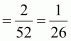1. Total number of face cards = 12

P (getting a face card)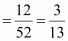1. Total number of red face cards = 6

P (getting a red face card)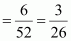1. Total number of Jack of hearts = 1

P (getting a Jack of hearts)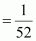1. Total number of spade cards = 13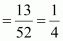1. Total number of queen of diamonds = 1

P (getting a queen of diamond)Question 15:

Five cards−−the ten, jack, queen, king and ace of diamonds, are well-shuﬄed with their face downwards. One card is then picked up at random.

1. What is the probability that the card is the queen?
2. If the queen is drawn and put aside, what is the probability that the second card picked up is (a) an ace? (b) a queen?

1. Total number of cards = 5

Total number of queens = 1

P (getting a queen)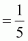1. When the queen is drawn and put aside, the total number of remaining cards will be 4.
1. Total number of aces = 1

P (getting an ace)

1. As queen is already drawn, therefore, the number of queens will be 0.

P (getting a queen)  = 0

Question 16:

12 defective pens are accidentally mixed with 132 good ones. It is not possible to just look at a pen and tell whether or not it is defective. One pen is taken out at random from this lot. Determine the probability that the pen taken out is a good one.

Total number of pens = 12 + 132 = 144

Total number of good pens = 132

P (getting a good pen)

Question 17:

1. A lot of 20 bulbs contain 4 defective ones. One bulb is drawn at random from the lot. What is the probability that this bulb is defective?
2. Suppose the bulb drawn in (i) is not defective and is not replaced. Now one bulb is drawn at random from the rest. What is the probability that this bulb is not defective?

1. Total number of bulbs = 20

Total number of defective bulbs = 4

P (getting a defective bulb)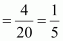1. Remaining total number of bulbs = 19

Remaining total number of non-defective bulbs = 16 − 1 = 15

P (getting a not defective bulb)

Question 18:

A box contains 90 discs which are numbered from 1 to 90. If one disc is drawn at random from the box, ﬁnd the probability that it bears

1. a two-digit number
2. a perfect square number
3. a number divisible by 5.

Total number of discs = 90

1. Total number of two-digit numbers between 1 and 90 = 81

P (getting a two-digit number)

1. Perfect squares between 1 and 90 are 1, 4, 9, 16, 25, 36, 49, 64, and 81. Therefore, total number of perfect squares between 1 and 90 is 9.

P (getting a perfect square)

1. Numbers that are between 1 and 90 and divisible by 5 are 5, 10, 15, 20, 25, 30, 35, 40, 45, 50, 55, 60, 65, 70, 75, 80, 85, and 90. Therefore, total numbers divisible by 5 = 18

Probability of getting a number divisible by 5

Question 19:

A child has a die whose six faces shows the letters as given below: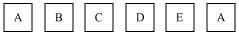The die is thrown once. What is the probability of getting (i) A? (ii) D?

Total number of possible outcomes on the dice = 6

1. Total number of faces having A on it = 2

P (getting A)

1. Total number of faces having D on it = 1

P (getting D)

Question 20:

Suppose you drop a die at random on the rectangular region shown in the given ﬁgure. What is the probability that it will land inside the circle with diameter 1 m?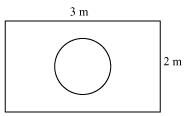Area of rectangle = l × b = 3 × 2 = 6 m2

Area of circle (of diameter 1 m)

P (die will land inside the circle)

Question 21:

A lot consists of 144 ball pens of which 20 are defective and the others are good. Nuri will buy a pen if it is good, but will not buy if it is defective. The shopkeeper draws one pen at random and gives it to her. What is the probability that

2. She will not buy it?

Total number of pens = 144

Total number of defective pens = 20

Total number of good pens = 144 − 20 = 124

1. Probability of getting a good pen

1. P (Nuri will not buy a pen)

Question 22:

Two dice, one blue and one grey, are thrown at the same time.

(i) Write down all the possible outcomes and complete the following table:

(ii) A student argues that ‘there are 11 possible outcomes 2, 3, 4, 5, 6, 7, 8, 9, 10, 11 and 12. Therefore, each of them has a probability . Do you agree with this argument?

1.    It can be observed that,

To get the sum as 2, possible outcomes = (1, 1)

To get the sum as 3, possible outcomes = (2, 1) and (1, 2)

To get the sum as 4, possible outcomes = (3, 1), (1, 3), (2, 2)

To get the sum as 5, possible outcomes = (4, 1), (1, 4), (2, 3), (3, 2)

To get the sum as 6, possible outcomes = (5, 1), (1, 5), (2, 4), (4, 2),

(3, 3)

To get the sum as 7, possible outcomes = (6, 1), (1, 6), (2, 5), (5, 2),

(3, 4), (4, 3)

To get the sum as 8, possible outcomes = (6, 2), (2, 6), (3, 5), (5, 3),

(4, 4)

To get the sum as 9, possible outcomes = (3, 6), (6, 3), (4, 5), (5, 4)

To get the sum as 10, possible outcomes = (4, 6), (6, 4), (5, 5)

To get the sum as 11, possible outcomes = (5, 6), (6, 5)

To get the sum as 12, possible outcomes = (6, 6)

1.     Probability of each of these sums will not be as these sums are not    equally likely.

Question 23:

A game consists of tossing a one rupee coin 3 times and noting its outcome each time. Hanif wins if all the tosses give the same result i.e., three heads or three tails, and loses otherwise. Calculate the probability that Hanif will lose the game.

The possible outcomes are

{HHH, TTT, HHT, HTH, THH, TTH, THT, HTT}

Number of total possible outcomes = 8

Number of favourable outcomes = 2 {i.e., TTT and HHH}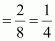P (Hanif will win the game)

P (Hanif will lose the game)

Question 24:

A die is thrown twice. What is the probability that:

1. 5 will not come up either time?
2. 5 will come up at least once?

[Hint: Throwing a die twice and throwing two dice simultaneously are treated as the same experiment].

Total number of outcomes = 6 × 6 = 36

1. Total number of outcomes when 5 comes up on either time are (5, 1), (5, 2), (5, 3), (5,4), (5, 5), (5, 6), (1, 5), (2, 5), (3, 5), (4, 5), (6, 5)

Hence, total number of favourable cases = 11

P (5 will come up either time)

P (5 will not come up either time)

1. Total number of cases, when 5 can come at least once = 11 P (5 will come at least once)

*Question 25:

Which of the following arguments are correct and which are not correct? Give reasons for your answer.

1. If two coins are tossed simultaneously there are three possible outcomes−−two heads, two tails or one of each. Therefore, for each of these outcomes, the probability is
2. If a die is thrown, there are two possible outcomes−−an odd number or an even number. Therefore, the probability of getting an odd number is

1. Incorrect

When two coins are tossed, the possible outcomes are (H, H), (H, T), (T, H), and (T, T). It can be observed that there can be one of each in two possible ways − (H, T), (T, H).

Therefore, the probability of getting two heads is , the probability of getting two tails is , and the probability of getting one of each is

It can be observed that for each outcome, the probability is not .

1. Correct

When a dice is thrown, the possible outcomes are 1, 2, 3, 4, 5, and 6. Out of these, 1, 3, 5 are odd and 2, 4, 6 are even numbers.

Therefore, the probability of getting an odd number is

Exercise 15.2

Question1:

Two customers Shyam and Ekta are visiting a particular shop in the same week (Tuesday to Saturday). Each is equally likely to visit the shop on any day as on another day. What is the probability that both will visit the shop on

(i) the same day (ii) consecutive days (iii) different days

There are a total of 5 days. Shyam can go to the shop in 5 ways and Ekta can go to the shop in 5 ways.

Therefore, total number of outcomes = 5 × 5 = 25

1. They can reach on the same day in 5 ways. i.e., (t, t), (w, w), (th, th), (f, f), (s, s)

P (both will reach on same day)

1. They can reach on consecutive days in these 8 ways – (t, w), (w, th), (th, f), (f, s), (w,t), (th, w), (f, th), (s, f).

Therefore, P (both will reach on consecutive days)

1. P (both will reach on same day) [(From (i)]

P (both will reach on different days)

Question 2:

A die is numbered in such a way that its faces show the number 1, 2, 2, 3, 3, 6. It is thrown two times and the total score in two throws is noted. Complete the following table which gives a few values of the total score on the two throws:

What is the probability that the total score is

(i) even? (ii) 6? (iii) at least 6?

Total number of possible outcomes when two dice are thrown = 6 × 6 = 36

1. Total times when the sum is even = 18

P (getting an even number)

1. Total times when the sum is 6 = 4

P (getting sum as 6)

1. Total times when the sum is at least 6 (i.e., greater than 5) = 15

P (getting sum at least 6)

*Question 3:

A bag contains 5 red balls and some blue balls. If the probability of drawing a blue ball is double that of a red ball, determine the number of blue balls in the bag.

Let the number of blue balls be x. Number of red balls = 5

Total number of balls = x + 5

P (getting a red ball)

P (getting a blue ball)

Given that,

However, the number of balls cannot be negative.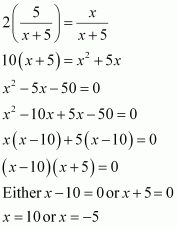Hence, number of blue balls = 10

Question 4:

A box contains 12 balls out of which x are black. If one ball is drawn at random from the box, what is the probability that it will be a black ball?

If 6 more black balls are put in the box, the probability of drawing a black ball is now double of what it was before. Find x.

Total number of balls = 12 Total number of black balls = x

P (getting a black ball)

If 6 more black balls are put in the box, then Total number of balls = 12 + 6 = 18

Total number of black balls = x + 6

P (getting a black ball now)

According to the condition given in the question,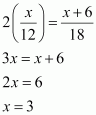*Question 5:

A jar contains 24 marbles, some are green and others are blue. If a marble is drawn at random from the jar, the probability that it is green is Find the number of blue balls in the jar.

Total number of marbles = 24

Let the total number of green marbles be x.

Then, total number of blue marbles = 24 – x

P (getting a given marble)

According to the condition given in the question,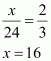Therefore, total number of green marbles in the jar = 16 Hence, total number of blue marbles

Chapter 15 of Class 10, Probability, belongs to the 6th Unit- Statistics and Probability, which adds up to 10 marks out of the total 80 marks in the examination. Unit 6 is one such unit, in which, marks can be easily scored once the method or the idea gets familiar. Solving the NCERT exercise questions will enable the students in getting thorough with the methods used in solving the problems of this chapter, in turn aiding the students to prepare well for the Class 10 Maths Board examination.
The main topics covered in this chapter include:
15.1 Introduction
15.2 Probability – A Theoretical Approach

List of Exercises in class 10 Maths Chapter 15
Exercise 15.1 Solutions 25 Questions (1 MCQ, 21 Short Answer Questions, 2  Long Answer Question, 1 Main Question with 4 Sub-questions)
Exercise 15.2 Solutions 5 Questions (5 Short Answer Questions)
Practising these NCERT Solutions for Class 10 is very important from the CBSE board examination point of view. Probability is one of the chapters in Class 10 maths that contain the concepts that are most likely to be used in daily life. The chapter deals with different topics related to the probability that includes:

1. The difference between experimental probability and theoretical probability
2. Why the probability of a sure event (or certain event) is 1.
3. How the probability of an impossible event is 0?
4. Elementary events.
5. Complementary events.
6. Finding the probability of different events.

### Key Features of NCERT Solutions for Class 10 Maths Chapter 15 – Probability

• In-depth knowledge of the chapter/problems is provided in easy language.
• Is based on the CBSE syllabus.
• Best study material to prepare for the Class 10 board exam.
• All the doubts that might arise while solving the exercise questions will vanish by going through these solutions

Conclusion The NCERT Solutions for Class 10 Maths Chapter 15 Probability provided by Swastik Classes are an excellent resource for students who want to understand the fundamental concepts of probability. Our expert team has created the solutions in a step-by-step approach to enable students to understand the concepts thoroughly.
Through our solutions, students can improve their problem-solving skills and prepare well for their board exams. These solutions are prepared based on the latest CBSE guidelines and are strictly based on the NCERT textbook.
We believe that our solutions will help students achieve their academic goals and pave the way for their success. We encourage students to use our solutions and strengthen their concepts in this chapter. With these solutions, students can develop a strong foundation in probability, which will be beneficial for their future studies and careers.

### Is NCERT Solutions for Class 10 Maths Chapter 15 important from exam point of view?

Yes, all the chapters of NCERT Solutions for Class 10 Maths Chapter 15 are important from an exam perspective. As this chapter explains probability which is important for board exams as well as for higher studies. The meaning of probability is basically the extent to which something is likely to happen. To find the probability of a single event to occur, first, we should know the total number of possible outcomes. So studying these probabilities makes learning easier to students. Most of the questions in exams come from this chapter.

### Give me a short summary on NCERT Solutions for Class 10 Maths Chapter 15?

NCERT Solutions for Class 10 Maths Chapter 15 has 2 exercises. The chapter deals with different topics related to the probability that includes:
1. The difference between experimental probability and theoretical probability
2. Why the probability of a sure event (or certain event) is 1.
3. How the probability of an impossible event is 0?
4. Elementary events.
5. Complementary events.
6. Finding the probability of different events.

### What are the key benefits of learning NCERT Solutions for Class 10 Maths Chapter 15?

The answers of NCERT Solutions for Class 10 Maths Chapter 15 have come in handy. This makes it easy to clear all the doubts related to probability. Easily solve all the questions easily which have been given for assignments by the class 10 students.